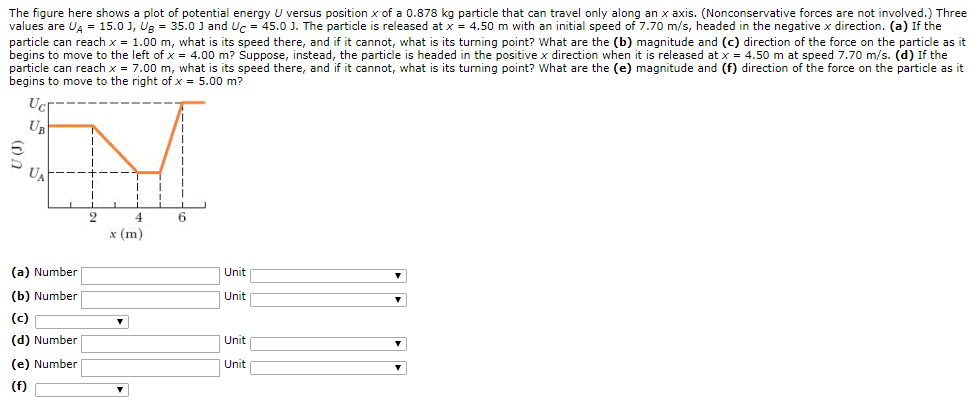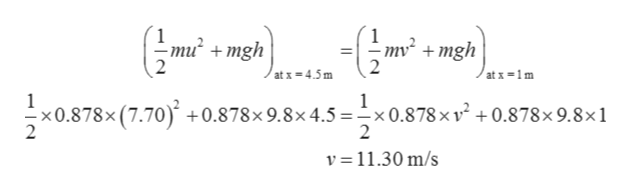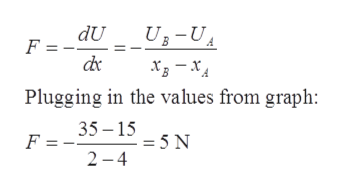# The figure here shows a plot of potential energy U versus position x of a 0.878 kg particle that can travel only along an x axis. (Nonconservative forces are not involved.) Threevalues are U = 15.0 J, Ug = 35.0 J and Uc 45.0 J. The particle is released at x = 4.50 m with an initial speed of 7.70 m/s, headed in the negative x direction. (a) If theparticle can reach x 1.00 m, what is its speed there, and if it cannot, what is its turning point? What are the (b) magnitude and (c) direction of the force on the particle as itbegins to move to the left of x = 4.00 m? Suppose, instead, the particle is headed in the positive x direction when it is released at x 4.50 m at speed 7.70 m/s. (d) If theparticle can reach x 7.00 m, what is its speed there, and if it cannot, what is its turning point? What are the (e) magnitude and (f) direction of the force on the particle as itbegins to move to the right of x 5.00 m?UBUA6(m)Unit(a) Number(b) NumberUnit(c)(d) Number|Unit(e) NumberUnit(f)(o

Question
3 viewshelp_outlineImage TranscriptioncloseThe figure here shows a plot of potential energy U versus position x of a 0.878 kg particle that can travel only along an x axis. (Nonconservative forces are not involved.) Three values are U = 15.0 J, Ug = 35.0 J and Uc 45.0 J. The particle is released at x = 4.50 m with an initial speed of 7.70 m/s, headed in the negative x direction. (a) If the particle can reach x 1.00 m, what is its speed there, and if it cannot, what is its turning point? What are the (b) magnitude and (c) direction of the force on the particle as it begins to move to the left of x = 4.00 m? Suppose, instead, the particle is headed in the positive x direction when it is released at x 4.50 m at speed 7.70 m/s. (d) If the particle can reach x 7.00 m, what is its speed there, and if it cannot, what is its turning point? What are the (e) magnitude and (f) direction of the force on the particle as it begins to move to the right of x 5.00 m? UB UA 6 (m) Unit (a) Number (b) Number Unit (c) (d) Number |Unit (e) Number Unit (f) (o fullscreen
check_circle

Step 1

(a)

Let the velocity at x = 1.0 m be v

We know:

Total energy = kinetic energy + potential energy

Step 2

From the conservation of energy:

(Total energy at x = 4.5 m) = (total energy at x = 1.0 m)help_outlineImage Transcriptionclose-om7 10.878x (7.70) +0.878x 9.8x4.5 =-x0.878 x v2 +0.878x 9.8x1 nu2 +mgh mv mgh atx 4.5m at x-1m 2 2 v 11.30 m/s fullscreen
Step 3

(b)

Let the magnitude of the force be F

Form...help_outlineImage TranscriptioncloseUB-U dU F = dr Х, — хд Plugging in the values from graph: 35 15 - 5 N 2 4 F = fullscreen

### Want to see the full answer?

See Solution

#### Want to see this answer and more?

Solutions are written by subject experts who are available 24/7. Questions are typically answered within 1 hour.*

See Solution
*Response times may vary by subject and question.
Tagged in

### Physics